# The mathematics of Feynman path integrals

Francesco de Vecchi (name.surname at uni-bonn.de)

Preliminary meeting: January 22nd, 10-12. R0.008

Seminar every Wednesday 12-14 N0.003 – Neubau

Feynman path integrals are a class of “infinite dimensional” oscillatory integrals which were introduced by R. Feynman in his reformulation of quantum mechanics. Instead of looking at the states of a quantum system as vectors in Hilbert space and at the related time-evolution, he introduced a space-time description of quantum evolution via classical trajectories weighted by complex valued phases. A mathematical formulation of his ideas has revealed itself to be quite challenging and many partial solutions to this problems have been considered.

In this seminar we will investigate some of the mathematical theories these objects, starting from the approach of Albeverio, Høeg-Krohn and Mazzucchi and possibly going to the coherent state approach of Klauder. Some application to quantum mechanical problems will be also discussed.

To fruitfully attend the seminar no previous knowledge of quantum mechanics is needed, on the other hand the seminar itself can be as an intuitive introduction of the phenomenology of quantum mechanics.

It is assumed some basic knowledge of functional analysis, especially Hilber space theory and spectral theory of bounded operators. Some probability/measure theory at the level of bachelor degree is also required. No stochastic calculus is necessary.

For an overview and historical introduction to Feynman integral see

Klauder, John R. “The Feynman Path Integral: An Historical Slice.” ArXiv:Quant-Ph/0303034, July 2003, 55–76. https://doi.org/10.1142/9789812795106_0005

References

• Mazzucchi, Sonia. Mathematical Feynman Path Integrals and Their Applications. Hackensack, NJ: World Scientific, 2009.

• Albeverio, Sergio A., Sonia Mazzucchi, and Raphael J. Høegh-Krohn. Mathematical Theory of Feynman Path Integrals: An Introduction. 2nd ed. Lecture Notes in Mathematics 523. Springer-Verlag Berlin Heidelberg, 2008.

• Klauder, John R. A Modern Approach to Functional Integration. 2011 edition. Berlin; New York: Birkhäuser, 2010.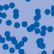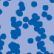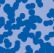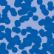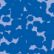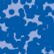## News

Der SFB 1060 Die Mathematik der emergenten Effekte hat eine dritte Förderperiode erhalten. (26.11.20)

Prof. Dr. Andreas Eberle erhält den diesjährigen Lehrpreis der Universität Bonn. (22.07.2020)

Herr Dr. Richard Höfer erhielt den Hausdorff-Gedächtnispreis 2019 der Fachgruppe Mathematik für die beste Disseration. Betreut wurde die Arbeit von Prof. J. Velázquez (29.01.2020).

Einer der Preise der Bonner Mathematischen Gesellschaft (BMG) für die besten Bachelorarbeiten 2019 ging an Herrn Peter Holderrieth unter der Betreuung von Prof. A. Eberle (29.01.2020).

Contact

Managing Director: Prof. Dr. Massimiliano Gubinelli